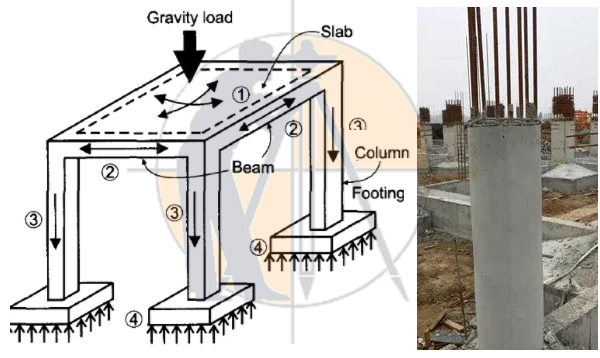Breaking News
Home / QA/QC Engineering / How To Calculate The Self Weight Of Column & Beam

# How To Calculate The Self Weight Of Column & Beam

## How To Calculate The Self Weight Of Column & Beam

Hello Guys in this topic we know about how to calculate self weight of column. In structural building designing by civil engineer it is considered that how much load is applied on column and distribute in footing and in soil by Foundation footing and column.

There is various types of load acting on RCC Column. Calculating the load applied on RCC column in structural designing and construction size of footing, size of column and size of beam will be decided according to strength capacity## Various types of load acting on column

1) Self weight of column

2) Self weight of beam per meter running length

3) Weight of slab per sq. meter

4) Brick wall per meter running length

### How to calculate self weight of column

Calculation :- height of column = 3 m

Assume Size of column = 9″×9″

Inch into mm = 230mm× 230mm

For calculating self load for column we have require self weight of concrete that is 2500 kg/m3 for RCC and self weight of Steel that is approx. 8000 kg/m3

Self weight of column is equal to weight of concrete in column and weight of Steel which is used in construction of column. First we calculate weight of concrete in column.

A) weight of concrete used in column

Size of column = 230mm×230mm

Height of column=3m

Volume of concrete = 0.23m×0.23m×3m

Volume of concrete= 0.1587 m3

Weight of concrete = volume × density

Weight of concrete =0.1587 m3×2500kg/m3

Weight of concrete = 396.75 kg

We should convert in kilo Newton we get load applied by concrete in column is about 3.96 kN

### How To Calculate The Tile Flooring Cost Per Square Feet

B) now calculate weight of Steel used in column by Thumb Rule we know that approx. 1% of concrete steel is used in column

Density of steel = 8000kg/m3

Volume of steel is 1% of concrete

Weight of steel = 0.01×0.1587m3×8000kg/m3

Weight of steel = 12.7 kg

Now convert kilo per meter into kilo Newton so load applied by Steel in column is approx. 0.127kN

Now total weight of column is equal to weight of concrete and weight of Steel

Total weight = 396.75 kg+12.7kg

Total weight = 409.446 kg

Now total self load of column is about 4.09 k.N acting on column

Hence total self load of column is about 4.09 kilo Newton per floor.

### THANKS.